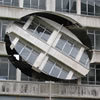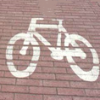#### You may also like### Turning the Place Over

As part of Liverpool08 European Capital of Culture there were a huge number of events and displays. One of the art installations was called "Turning the Place Over". Can you find our how it works?### Where Art and Maths Combine

In this article, Rachel Melrose describes what happens when she mixed mathematics with art.### It Depends on Your Point of View!

Anamorphic art is used to create intriguing illusions - can you work out how it is done?

# Moving Squares

##### Age 14 to 16Challenge Level

Robert from Bishop Tonnos High School in Canada sent us the following solution:

In the picture viewing the cylinders from above, take each line that forms a radius of the circle, and draw a horizontal line between the left edge of the page and its contact point on the circle. The radial divisions of the circle are equal; we'll call this angle $\theta$.

Radius of the circle is $R$, and square size of the $n^{th}$ square will be denoted $S_n$.

It's clear that for the first square, $S_1 = R\sin\theta$.

For the second square, $S_2 = R\sin(2\theta)-R\sin\theta$

What this problem reduces to is finding the difference between horizontal lines, since it is the "overhang" which will determine the apparent size of a square. The difference between lines (and therefore the width of each square) is:

$$S_n=R\sin(n\theta)-R\sin((n-1)\theta)$$

Louis from Eltham College considered what would happen with a cylinder with $n$ divisions around it. You can read his solution here.